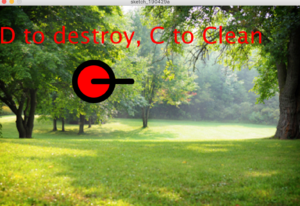# Serial Communication : Save Our Planet

I wanted to make something with a message towards good and what is in dire need of immediate good treatment and aid? Our planet.

Arduino Code:

```#include <Servo.h>

Servo myservo;

int pos = 0;
int ServoPin = 10;
int ServoAngle;

void setup() {
// start serial port at 9600 bps:
Serial.begin(9600);
myservo.attach(ServoPin);
}

void loop() {
if (Serial.available() > 0) {
myservo.write(ServoAngle);
}
}```

Processing Code:

```int new_angle, this_angle, temp_angle;
int e_rad = 100, e_ctr_x = 250, e_ctr_y = 200;
int l_length = 100;
int r_ctr_x = e_ctr_x, r_ctr_y = e_ctr_y;
color angle_color = 200;
PImage bg;

Serial port;
Serial type;

void setup() {

size(800, 533);
new_angle = 90;
printArray(Serial.list());
String portname=Serial.list();
println(portname);
port = new Serial(this,portname,9600);
this_angle = 90;
strokeWeight(15);
}

void draw() {
background(bg);
textSize(60);
text("D to destroy, C to Clean" ,0,100);
if (this_angle < new_angle) {
//println("1) ", new_angle, angle);
this_angle = this_angle + 5;
}
if (this_angle > new_angle) {
//println("2) ", new_angle, angle);
this_angle = this_angle - 5;
}
angle_color = int((float(this_angle)/180) * 255);
fill(255, angle_color, 0);
//calculate the outer point of the lever arm
int x2 = int(cos(radians(this_angle)) * l_length + e_ctr_x);
int y2 = int(sin(radians(this_angle)) * l_length + e_ctr_y);
//println(x2, y2);
line(e_ctr_x, e_ctr_y, x2, y2);

if (temp_angle != this_angle) {
port.write(this_angle);  //write the servo angle to the serial port
temp_angle = this_angle;
println(this_angle);
}
}
void keyPressed(){
switch(key) {
case 'd':
new_angle = 0;
break;
case 'c':
new_angle = 180;
break;
}
//println(new_angle);
}```

This is what the interface looks like on the computer (the processing screen):

When you click C, the servo turns to the clean ocean, full of animals and plants and the text turns to yellow. When you click D, the servo turns to the polluted ocean scene and the text turns to red.